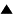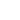# Neutral evolution game

Neutral selection plays an important role in the evolution of populations, having a finite population size n  . To demonstrate the neutral selection features explicitly, let's consider the pure neutral evolution game, which is defined as follows:
 ; 1. There is a population of black and white balls, the total number of the balls in a population is equal to n . ; 2. The evolution consists of consequent generations. Each generation consists of two steps. At the first step we duplicate each ball, conserving its color: a black ball has two black offsprings, a white ball has two white ones. At the second step we randomly remove from a population exactly half of the balls with equal probability for black and white "species", independently of their color.

We say that the population is in l -state, if the numbers of black and white balls at a considered generation are equal to l and n-l, respectively. We can characterize the evolution by the probability Plm of a transition from l -state to m -state during one generation. Using a straightforward combinatorial consideration, we can calculate the values of Plm :The matrix Plm determines the random Markovian process, which can be considered as an example of a simple stochastic genetic process . Using the general methods of analysis of such processes , we can deduce that:

1) the considered process always converges to one of two absorbing states, namely, to 0-state (all balls are white), either to n-state (all balls are black);

2) at large n the characteristic number of generations Tn , needed to converge to the either absorbing state, is equal to 2n :
 Tn = 2n . (2)

Thus, although this evolution is purely neutral (black and white balls have equal chances to survive), nevertheless only one species is selected. The value Tn characterizes the neutral selection rate, it is used in our estimations (Quasispecies , Estimation of the evolution rate).

References:

1. M. Kimura. "The neutral theory of molecular evolution". Cambridge Un-ty Press. 1983.

2. S. Karlin. "A first course in stochastic processes". Academic Press. New York, London, 1968.

 HomeMetasystem Transition TheoryEvolutionary TheoryMathematical Modeling of EvolutionModels of molecular-genetic systems originQuasispecies Up Prev.Next Down